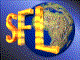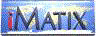| iMatix home page | << | < | > | >>SFLVersion 2.11

### cgi_fld_len_by_index

```#include "sflhttp.h"
int
cgi_fld_len_by_index (
int iPos,
char *strIn,
int *iDataLen,
int *iNameLen)
```

#### Synopsis

Gets the length of the field data from a CGI input stream based off a field index. The index is from 0 to n. Where 0 would be the first field in the stream, and n would be the last. example: Form snippet: Product <INPUT TYPE="text" NAME="SENDERID" SIZE=80 VALUE="mailto"> Version <INPUT TYPE="text" NAME="VERSION" SIZE=80 VALUE="v1.4"> E-mail <INPUT TYPE="text" NAME="Email" SIZE=80> CGI code to get form field: cgi fld len by index (1, strStdin, &iDataLen, &iNameLen); On return from the call: iDataLen would = 4 and iNameLen would be = 7 Submitted by Scott Beasley jscottb@infoave.com

#### Source Code - (sflhttp.c)

```{
int iLen;
char *strTmp;

ASSERT (strIn);

iLen = getstrfldlen (strIn, iPos, 0, "&");

if (iLen)
{
strTmp = (char *) malloc (sizeof (char) * iLen + 1);

getstrfld (strIn, iPos, 0, "&", strTmp);
http unescape (strTmp, NULL);
iLen = getstrfldlen (strTmp, 1, 0, "=");
if (iDataLen)
*iDataLen = iLen;

if (iNameLen)
*iNameLen = getstrfldlen (strTmp, 0, 0, "=");

free (strTmp);
}

return iLen;
}
```

 | << | < | > | >>Copyright © 1996-2000 iMatix Corporation